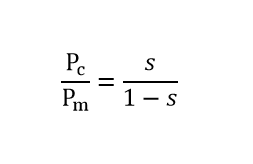# Relationship between Rotor Input, Copper Loss and mechanical power

### Rotor Input vs Copper Loss vs mechanical power;

The rotor input power P2, rotor copper loss Pc, gross mechanical power developed Pm and slip has below-mentioned relation.

P2 : Pc : Pm = 1 : s : (1-s)

From that,

P2 – Pc = Pm

(1-s) P2 = Pm

Pc = s P2Using the formula,

1. The total mechanical power is developed in the rotor is equal to the (1-s) times of the rotor input.
2. The total copper loss is equal to the slip s times of the rotor input.
3. Total mechanical power is equal to the difference between rotor input to rotor copper loss.

The above relation is used to find out the solution for power flow diagrams.